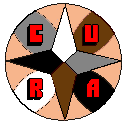The Manifesto | The 8 Houses | Texts and Articles | Review | Links | ACCUEIL (FR) | HOME (EN)

 Zodiac based on a different Plane by Slawomir Stachniewicz

Perhaps each astrologer knows very well what is the Zodiac: a circle of 12 signs each 30° wide in the plane of the Earth's orbit, starting from the point of the vernal equinox also known as the Point of Aries. One may ask a question: what would the Zodiac look like if it was based on a different plane, e.g. a plane of some other planet's orbit. To answer this question I will need to introduce some mathematics. I think the easiest way to imagine and calculate that is to use vectors.

What is a vector? It is a mathematical object characterized by three quantities: direction, length and orientation. E.g. if we have two points and if we agree that one is the beginning and the other is the end, they determine a vector: its direction is determined by the line connecting both points, length is equal to the distance between them and orientation is simply from the beginning to the end. If we are in three-dimensional (3D) space and if we introduce a rectangular system of coordinates, a vector may be described by three numbers called components. It is also possible to use non-rectangular systems of coordinates and more dimensions but in such systems things get more complicated so we will restrict us to rectangular coordinates and three dimensions.

Apart from vectors, there are also similar objects called pseudovectors. Their properties are almost the same but their orientation is handled in a different way: it is described by direction of rotation. We may describe pseudovectors like vectors (e.g. by providing three components) but we need to choose a convention: whether clockwise rotation is associated with orientation from the object to the observer or it is opposite, usually we use the second one. E.g. if we take the Earth's rotation around its axis and if we apply this convention, the pseudovector of its angular speed is orientated from the South to the North. Of course, its direction is parallel to the North-South axis and its length is equal to the value of the angular speed. This convention is called the left-hand rule: if we rotate fingers and the rest of the left hand in the horizontal plane anticlockwise, the thumb points up.

If we have two vectors or pseudovectors, we may calculate their scalar and vector products. Scalar product is a number and it is equal to product of lengths of both (pseudo)vectors and cosine of angle between them. Scalar product of two vectors or two pseudovectors is a scalar, so its sign does not depend on convention, scalar product of a vector and pseudovector is a pseudoscalar, so its sign depends on convention. If we swap both (pseudo)vectors, the result is the same. Vector product is a vector or a pseudovector. Its length is equal to product of lengths of both (pseudo)vectors and sine of angle between them, its direction is perpendicular to both (pseudo)vectors and its orientation is determined by the left hand rule (we rotate left hand from the first vector to the other). Vector product of two vectors or two pseudovectors is a pseudovector, vector product of a vector and pseudovector is a vector. If we swap both (pseudo)vectors, the result is opposite. Scalar product of two perpendicular vectors is zero, vector product of two parallel vectors is zero.

In order to make life easier, we will neglect the difference between  vectors and pseudovectors, scalars and pseudoscalars so we will speak about vectors and scalars only. Moreover, we will mark scalars with normal letters and vectors with bold letters, scalar product of two vectors A and B by A·B and their vector product by A×B. It is quite easy to prove that

A·(B+C) = A·B + A·C, (A+B)·C = A·C + B·C
A×(B+C) = A×B + A×C, (A+B)×C = A×C + B×C

Scalar and vector products also have some other interesting properties, e.g.

A·(B×C) = B·(C×A) = C·(A×B)
(A×B)·C = (B×C)·A = (C×A)·B
A×(B×C) = (A·C)B - (A·B)C
(A×B)·(C×D) = (A·C)(B·D) + (A·D)(B·C)

Now let us introduce a system of rectangular coordinates. Let us call the first coordinate x, the second y and the third z and we arrange them in a way that

x×y = z (y×x = -z)
y×z = x (z×y = -x)
z×x = y (x×z = -y)

where x, y and z are base vectors. They are perpendicular to each other and their length is equal to 1 so they are unit vectors.

Now we will show how to describe any vector by its components. E.g. if we have a vector a, it is a sum of

a = ax x + ay y + az z

where components ax, ay, and az are unique or, in other words, none of the other three numbers would fulfill the above condition (it is a consequence of the fact that we have chosen a 3D space and none of the base vector may be expressed as a linear combination of the others). Sometimes it is convenient to write it in a form of a matrix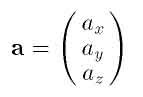If we use a system of rectangular coordinates as described, scalar product of two vectors a and b is equal to sum of products of all components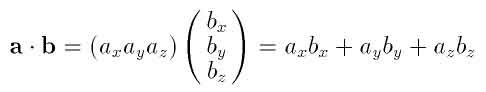and their vector product is equal to the determinant of a matrix that consists of components of both vectors and all three base vectors: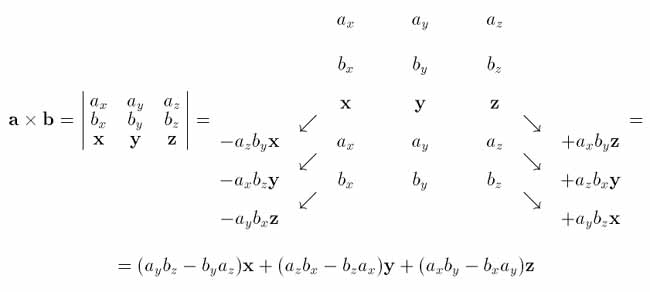When describing rectangular system of coordinates, there were mentioned unit vectors. Unit vector is a vector of length equal to 1. Length of other vectors is a square root of a scalar product of this vector with itself: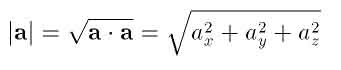If we have a vector and we need an unit vector in the same direction, it is enough to divide the vector by its length.

Now we are ready to describe the Zodiac in terms of vectors. Zodiac is determined by two Earth's motions: rotation around its axis and orbital motion around the Sun (or, more precisely, around the centre of mass of the Solar System). Let us write vectors of both angular speeds as w and W. It is obvious that both vectors point to north poles of the equatorial and ecliptic systems, respectively. If we take a vector product of w and W

¡ = w×W

we will obtain a vector that points in the direction of the Point of Aries. In general, it is a non-unit vector.

Now let us think about spherical coordinates. Astrologers usually use ecliptic coordinates, where the first coordinate is ecliptic longitude l and the second one is ecliptic latitude b. Because astrologers deal with objects at the celestial sphere, it is enough and it is not necessary to take care about the distance. Usually it is assumed that all objects are infinitely far, for convenience we will assume that the distance to celestial sphere is equal to 1. Third coordinate often used by astrologers, declination d, is a part of some other system called equatorial. It is based on the plane of the Earth's (and celestial) equator, it starts in the Point of Aries and declination is the second coordinate, the first one is right ascension a. Of course, we may express all these coordinates using vectors.

Let us take an object at the celestial sphere. Let us write the vector from the observer to this object as R. Let us remember that because we assume that the distance to celestial sphere is 1, it is an unit vector. Let us assume that both vectors w and W are also unit ones - simply we redefine them as vectors from observer to the Earth's and ecliptic poles. A projection of R to the ecliptic plane is equal to

Rp = R - W(R·W)

Then we may calculate ecliptic latitude

cos b = |Rp|, sin b = R·W

and longitude

sin l = W·(¡×Rp)/(|¡||Rp|) = ¡·(Rp×W)/(|¡||Rp|) = ¡·(R×W)/(|¡||Rp|) =
= (w×W)·(R×W)/(|w×W| cos b)

so

sin l cos b = (w×W)·(R×W) / |w×W| = [(w·R)(W·W) - (w·W)(W·R)] / [w2W2 - (w·W)2]1/2 =
= (w·R - sin bw·W) / [1 - (w·W)2]1/2 = (w·R - sin b cos e) / sin e

where e is the inclination of ecliptic to equator (in other words, it is the angle between w and W). We may also write an equation for cosine of the ecliptic longitude

cos l = ¡·Rp/|¡||Rp| = (w×W)·[R - W(R·W)] / (|w×W| cos b) = (w×W)·R / (|w×W| cos b)

so

cos l cos b = (w×W)·R / |w×W| = (w×W)·R / sin e

Now let us take an orbit of some other planet. Let us write the vector from the observer to the north pole of the orbit as Wp. Usually orbital planes are described by two angles: ascending node N (usually marked with a symbol similar to W) and inclination i. Let us define vector N as the vector from the observer to the ascending node of the orbit. It is quite easy to prove that

W×Wp = N sin i
W·Wp = cos i

From the first equation we can obtain Wp. Let us take a vector product of W with both sides of the equation

W×(N sin i) = W×(W×Wp) = (W·Wp)W - W2Wp = W cos i - Wp

so

Wp = W cos i - (W×N) sin i = W cos i + (N×W) sin i

Once we have Wp and we decide to keep the Earth's equator, we can build new Zodiac based on a new ecliptic plane. Everything we need is to replace W with Wp:

sin bp = R·Wp
sin lp cos bp = (w·R - sin bp cos ep) / sin ep
cos lp cos bp = (w×Wp)·R / sin ep

where cos ep = w·Wp and sin ep = (1 - cos2ep)1/2

What should we do now is to set some rectangular coordinates. As astrologers use ecliptic coordinates, the most natural one would be based on the ecliptic and the vernal equinox point. For example, the x axis could be the axis between the vernal and autumn equinox points, y axis between the winter and summer solstice points and z axis between south and north ecliptic poles. Unit vectors x, y and z would be defined in the following way:

• x would be the vector between the observer and the vernal equinox point (or the beginning of the sign of Aries)
• y would be the vector between the observer and the summer solstice point (or the beginning of the sign of Cancer)
• z would be the vector between the observer and the north ecliptic pole.
If an object at the celestial sphere has ecliptic longitude l and ecliptic latitude b, its rectangular coordinates are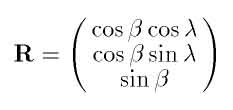(R is the vector between the observer and the object). Using this formula, we may determine coordinates of vectors w, W and N: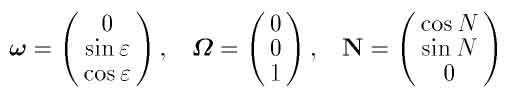Given that, we can calculate Wp (we remember that Wp =W cos i + (N×W) sin i):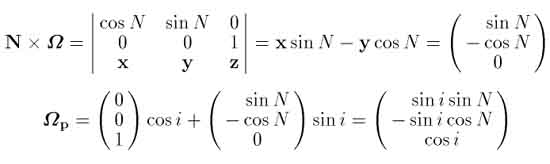Finally, there is perhaps the most boring part of work: to take these vectors, put into formulas and calculate new spherical coordinates lp and bp. First of all bp:

sin bp = R·Wp = (sin i sin N cos b cos l - sin i cos N cos b sin l + sin b cos i) = [sin i cos b (sin N cos l - cos N sin l) + sin b cos i] = [sin b cos i - sin i cos b sin (l-N)]

then ep:

cos ep = w·Wp = cos e cos i - cos N sin e sin i

and, finally, lp (it will be the most complicated part of work):

sin lp cos bp = (w·R - sin bp cos ep) / sin ep
sin lp cos bp sin ep = (w·R - sin bp cos ep) = sin e cos b  sin l + cos e sin b - sin bp cos ep

cos lp cos bp = (w×Wp)·R / sin ep
cos lp cos bp sin ep = (w×Wp)·R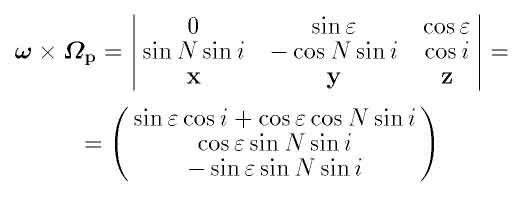hence we have

cos lp cos bp sin ep = (sin e cos i + cos e cos N sin i) cos b cos l + cos e sin N sin i cos b sin l - sin e sin N sin i sin b

If we wanted to change equator to the planet's one, we would need to know its orbital elements Np and ip referred to the ecliptic and, knowing them, to calculate wp in a way similar to Wp and to put wp instead of w. Of course, final formulas for new spherical coordinates would be much more complicated.

As we can see, vectors are very convenient when deriving formulas in spherical astronomy. While final formulas are the same, one can avoid lenghty ones in the middle. Moreover, they are useful when constructing various objects. Maybe astrologers should pay more attention to them.

Reference of the page: